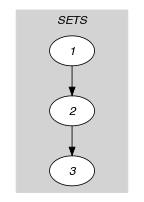## 5.210. k_same_partition

Origin
Constraint

$𝚔_\mathrm{𝚜𝚊𝚖𝚎}_\mathrm{𝚙𝚊𝚛𝚝𝚒𝚝𝚒𝚘𝚗}\left(\mathrm{𝚂𝙴𝚃𝚂},\mathrm{𝙿𝙰𝚁𝚃𝙸𝚃𝙸𝙾𝙽𝚂}\right)$

Types
 $\mathrm{𝚅𝙰𝚁𝙸𝙰𝙱𝙻𝙴𝚂}$ $\mathrm{𝚌𝚘𝚕𝚕𝚎𝚌𝚝𝚒𝚘𝚗}\left(\mathrm{𝚟𝚊𝚛}-\mathrm{𝚍𝚟𝚊𝚛}\right)$ $\mathrm{𝚅𝙰𝙻𝚄𝙴𝚂}$ $\mathrm{𝚌𝚘𝚕𝚕𝚎𝚌𝚝𝚒𝚘𝚗}\left(\mathrm{𝚟𝚊𝚕}-\mathrm{𝚒𝚗𝚝}\right)$
Arguments
 $\mathrm{𝚂𝙴𝚃𝚂}$ $\mathrm{𝚌𝚘𝚕𝚕𝚎𝚌𝚝𝚒𝚘𝚗}\left(\mathrm{𝚜𝚎𝚝}-\mathrm{𝚅𝙰𝚁𝙸𝙰𝙱𝙻𝙴𝚂}\right)$ $\mathrm{𝙿𝙰𝚁𝚃𝙸𝚃𝙸𝙾𝙽𝚂}$ $\mathrm{𝚌𝚘𝚕𝚕𝚎𝚌𝚝𝚒𝚘𝚗}\left(𝚙-\mathrm{𝚅𝙰𝙻𝚄𝙴𝚂}\right)$
Restrictions
 $\mathrm{𝚛𝚎𝚚𝚞𝚒𝚛𝚎𝚍}$$\left(\mathrm{𝚅𝙰𝚁𝙸𝙰𝙱𝙻𝙴𝚂},\mathrm{𝚟𝚊𝚛}\right)$ $|\mathrm{𝚅𝙰𝚁𝙸𝙰𝙱𝙻𝙴𝚂}|\ge 1$ $|\mathrm{𝚅𝙰𝙻𝚄𝙴𝚂}|\ge 1$ $\mathrm{𝚛𝚎𝚚𝚞𝚒𝚛𝚎𝚍}$$\left(\mathrm{𝚅𝙰𝙻𝚄𝙴𝚂},\mathrm{𝚟𝚊𝚕}\right)$ $\mathrm{𝚍𝚒𝚜𝚝𝚒𝚗𝚌𝚝}$$\left(\mathrm{𝚅𝙰𝙻𝚄𝙴𝚂},\mathrm{𝚟𝚊𝚕}\right)$ $\mathrm{𝚛𝚎𝚚𝚞𝚒𝚛𝚎𝚍}$$\left(\mathrm{𝚂𝙴𝚃𝚂},\mathrm{𝚜𝚎𝚝}\right)$ $|\mathrm{𝚂𝙴𝚃𝚂}|>1$ $\mathrm{𝚜𝚊𝚖𝚎}_\mathrm{𝚜𝚒𝚣𝚎}$$\left(\mathrm{𝚂𝙴𝚃𝚂},\mathrm{𝚜𝚎𝚝}\right)$ $\mathrm{𝚛𝚎𝚚𝚞𝚒𝚛𝚎𝚍}$$\left(\mathrm{𝙿𝙰𝚁𝚃𝙸𝚃𝙸𝙾𝙽𝚂},𝚙\right)$ $|\mathrm{𝙿𝙰𝚁𝚃𝙸𝚃𝙸𝙾𝙽𝚂}|\ge 2$
Purpose

Given a collection of $|\mathrm{𝚂𝙴𝚃𝚂}|$ sets, each containing the same number of domain variables, the $𝚔_\mathrm{𝚜𝚊𝚖𝚎}_\mathrm{𝚙𝚊𝚛𝚝𝚒𝚝𝚒𝚘𝚗}$ constraint forces a $\mathrm{𝚜𝚊𝚖𝚎}_\mathrm{𝚙𝚊𝚛𝚝𝚒𝚝𝚒𝚘𝚗}$ constraint between each pair of consecutive sets.

Example
$\left(\begin{array}{c}〈\begin{array}{c}\mathrm{𝚜𝚎𝚝}-〈1,2,6,3,1,2〉,\hfill \\ \mathrm{𝚜𝚎𝚝}-〈6,6,2,3,1,3〉,\hfill \\ \mathrm{𝚜𝚎𝚝}-〈2,2,2,1,1,1〉\hfill \end{array}〉,\hfill \\ 〈𝚙-〈1,3〉,𝚙-〈4〉,𝚙-〈2,6〉〉\hfill \end{array}\right)$

The first argument $\mathrm{𝚂𝙴𝚃𝚂}$ of the $𝚔_\mathrm{𝚜𝚊𝚖𝚎}_\mathrm{𝚙𝚊𝚛𝚝𝚒𝚝𝚒𝚘𝚗}$ constraint corresponds to 3 collections of variables, while the second argument $\mathrm{𝙿𝙰𝚁𝚃𝙸𝚃𝙸𝙾𝙽𝚂}$ defines the 3 sets of values $\left\{1,3\right\}$, $\left\{4\right\}$ and $\left\{2,6\right\}$. The $𝚔_\mathrm{𝚜𝚊𝚖𝚎}_\mathrm{𝚙𝚊𝚛𝚝𝚒𝚝𝚒𝚘𝚗}$ constraint holds since:

• The first and second collections of variables are assigned 3 values in the $\left\{1,3\right\}$ as well as 3 values in $\left\{2,6\right\}$.

• The second and third collections of variables are also assigned 3 values in the $\left\{1,3\right\}$ as well as 3 values in $\left\{2,6\right\}$.

Typical
$|\mathrm{𝚅𝙰𝚁𝙸𝙰𝙱𝙻𝙴𝚂}|>1$
Symmetries
• Items of $\mathrm{𝚂𝙴𝚃𝚂}$ are permutable.

• Items of $\mathrm{𝚂𝙴𝚃𝚂}.\mathrm{𝚜𝚎𝚝}$ are permutable.

• Items of $\mathrm{𝙿𝙰𝚁𝚃𝙸𝚃𝙸𝙾𝙽𝚂}$ are permutable.

• Items of $\mathrm{𝙿𝙰𝚁𝚃𝙸𝚃𝙸𝙾𝙽𝚂}.𝚙$ are permutable.

• An occurrence of a value of $\mathrm{𝚂𝙴𝚃𝚂}.\mathrm{𝚜𝚎𝚝}.\mathrm{𝚟𝚊𝚛}$ can be replaced by any other value that also belongs to the same partition of $\mathrm{𝙿𝙰𝚁𝚃𝙸𝚃𝙸𝙾𝙽𝚂}$.

Arg. properties

Contractible wrt. $\mathrm{𝚂𝙴𝚃𝚂}$.

Keywords
Arc input(s)

$\mathrm{𝚂𝙴𝚃𝚂}$

Arc generator
$\mathrm{𝑃𝐴𝑇𝐻}$$↦\mathrm{𝚌𝚘𝚕𝚕𝚎𝚌𝚝𝚒𝚘𝚗}\left(\mathrm{𝚜𝚎𝚝}\mathtt{1},\mathrm{𝚜𝚎𝚝}\mathtt{2}\right)$

Arc arity
Arc constraint(s)
$\mathrm{𝚜𝚊𝚖𝚎}_\mathrm{𝚙𝚊𝚛𝚝𝚒𝚝𝚒𝚘𝚗}$$\left(\mathrm{𝚜𝚎𝚝}\mathtt{1}.\mathrm{𝚜𝚎𝚝},\mathrm{𝚜𝚎𝚝}\mathtt{2}.\mathrm{𝚜𝚎𝚝},\mathrm{𝙿𝙰𝚁𝚃𝙸𝚃𝙸𝙾𝙽𝚂}\right)$
Graph property(ies)
$\mathrm{𝐍𝐀𝐑𝐂}$$=|\mathrm{𝚂𝙴𝚃𝚂}|-1$

Graph model

Parts (A) and (B) of Figure 5.210.1 respectively show the initial and final graph associated with the Example slot. To each vertex corresponds a collection of variables, while to each arc corresponds a $\mathrm{𝚜𝚊𝚖𝚎}_\mathrm{𝚙𝚊𝚛𝚝𝚒𝚝𝚒𝚘𝚗}$ constraint.

##### Figure 5.210.1. Initial and final graph of the $𝚔_\mathrm{𝚜𝚊𝚖𝚎}_\mathrm{𝚙𝚊𝚛𝚝𝚒𝚝𝚒𝚘𝚗}$ constraint(a) (b)